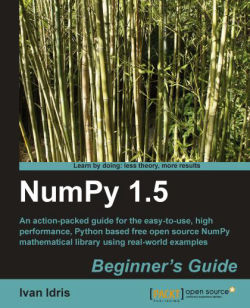### NumPy 1.5 Beginner's Guide5 (1 reviews total)
By Ivan Idris

• Constantly updated with 100+ new titles each month
• Breadth and depth in over 1,000+ technologies

In today's world of science and technology, the hype is all about speed and flexibility. When it comes to scientific computing, NumPy is on the top of the list. NumPy is the fundamental package needed for scientific computing with Python. NumPy will give you both speed and high productivity. Save thousands of dollars on expensive software, while keeping all the flexibility and power of your favourite programming language.

NumPy 1.5 Beginner's Guide will teach you about NumPy from scratch. It includes everything from installation, functions, matrices, and modules to testing, all explained with appropriate examples.

Numpy 1.5 Beginner's Guide will teach you about installing and using NumPy and related concepts.

This book will give you a solid foundation in NumPy arrays and universal functions. At the end of the book, we will explore related scientific computing projects such as Matplotlib for plotting and the SciPy project through examples.

NumPy 1.5 Beginner's Guide will help you be productive with NumPy and write clean and fast code.

Publication date:
November 2011
Publisher
Packt
Pages
234
ISBN
9781849515306

## Chapter 1. NumPy Quick Start

Let's get started. We will install NumPy on different operating systems and have a look at some simple code that uses NumPy. The IPython interactive shell is introduced briefly. As mentioned in the preface, SciPy is closely related to NumPy, so you will see the SciPy name appearing here and there. At the end of this chapter, you will find pointers on how to find additional information online if you get stuck or are uncertain about the best way to solve problems.

In this chapter, we shall:

• Install Python and NumPy on Windows

• Install Python and NumPy on Linux

• Install Python and NumPy on Macintosh

• Write simple NumPy code

• Get to know IPython

• Browse online documentation and resources

## Python

NumPy is based on Python, so it is required to have Python installed. On some operating systems, Python is already installed. You, however, need to check whether the Python version corresponds with the NumPy version you want to install.

## Time for action – installing Python on different operating systems

NumPy has binary installers for Windows, various Linux distributions and Mac OS X. There is also a source distribution, if you prefer that. You need to have Python 2.4.x or above installed on your system. We will go through the various steps required to install Python on the following operating systems:

1. Debian and Ubuntu : Python might already be installed on Debian and Ubuntu but the development headers are usually not. On Debian and Ubuntu install python and python-dev with the following commands:

```sudo apt-get install python
sudo apt-get install python-dev```
2. Windows : The Windows Python installer can be found at www.python.org/download. On this website, we can also find installers for Mac OS X and source tarballs for Linux, Unix, and Mac OS X.

3. Mac: Python comes pre-installed on Mac OS X . We can also get Python through MacPorts, Fink, or similar projects.

We can install, for instance, the Python 2.6 port by running the following command:

`sudo port install python26`

`LAPACK` does not need to be present but, if it is, NumPy will detect it and use it during the installation phase. It is recommended to install `LAPACK` for serious numerical analysis.

### What just happened?

We installed Python on Debian, Ubuntu, Windows, and the Mac.

### Tip

You can download the example code files for all Packt books you have purchased from your account at http://www.PacktPub.com. If you purchased this book elsewhere, you can visit http://www.PacktPub.com/support and register to have the files e-mailed directly to you.

## Windows

Installing NumPy on Windows is straightforward. You only need to download an installer, and a wizard will guide you through the installation steps.

## Time for action – installing NumPy on Windows

Installing NumPy on Windows is necessary but, fortunately, a straightforward task. The actions we will take are as follows: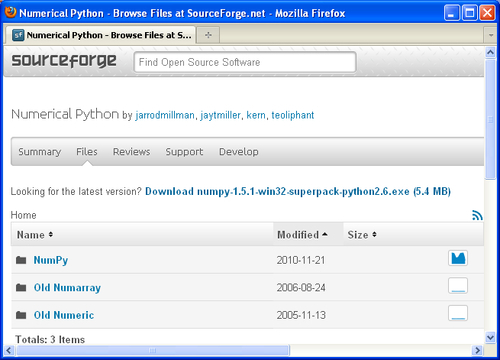Choose the appropriate version. In this example, we chose `numpy-1.5.1-win32-superpack-python2.6.exe`.

2. Open the installer: Open the EXE installer by double clicking on it.

3. NumPy features: Now, we see a description of NumPy and its features. Click Next.

4. Install Python: If you have Python installed, it should automatically be detected. If it is not detected, maybe your path settings are wrong. At the end of this chapter, resources are listed in case you have problems with installing NumPy: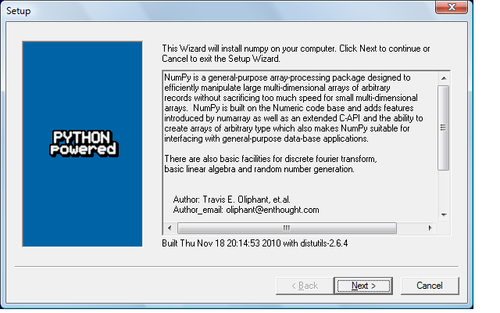5. Finish the installation: In this example, Python 2.6 was found. Click Next if Python is found; otherwise, click Cancel and install Python (NumPy cannot be installed without Python). Click Next. This is the point of no return. Well, kind of, but it is best to make sure that you are installing to the proper directory and so on and so forth. Now the real installation starts. This may take a while: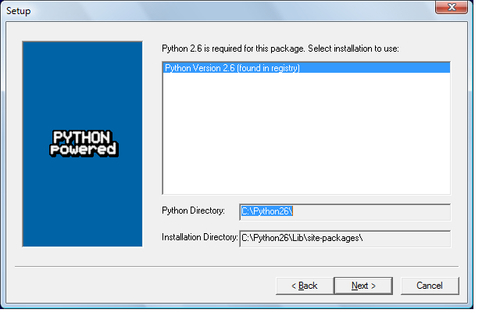### What just happened?

We installed NumPy on Windows.

## Linux

Installing NumPy on Linux depends on the distribution you have. We will discuss how you would install NumPy from the command line, although you could probably use graphical installers; it depends on your distribution (distro).

## Time for action – installing NumPy on Linux

Most Linux distributions have NumPy packages. We will go through the necessary steps for some of the popular Linux distros:

1. Installing NumPy on Red Hat: Run the following instructions from the command line:

`yum install python-numpy`
2. Installing NumPy on Mandriva : To install NumPy on Mandriva, run the following command line instruction:

`urpmi python-numpy`
3. Installing NumPyon Gentoo : To install NumPy on Gentoo run the following command line instruction:

`sudo emerge numpy`
4. Installing NumPyon Debian andUbuntu : On Debian or Ubuntu, we need to type the following:

`sudo apt-get install python-numpy`

The following table gives an overview of the Linux distributions and corresponding NumPy package names.

Linux distribution

Package name

Arch Linux

python-numpy

Debian

python-numpy

Fedora

numpy

Gentoo

dev-python/numpy

OpenSUSE

python-numpy, python-numpy-devel

Slackware

numpy

### What just happened?

We installed NumPy on various Linux distributions.

## Mac OS X

You can install NumPy on the Mac with a graphical installer or from the command-line from a port manager such as MacPorts or Fink, depending on your preference.

## Time for action – installing NumPy on Mac OS X with a GUI installer

We will install NumPy with a GUI installer.

1. Download the GUI installer: We can get a NumPy installer from the SourceForge website http://sourceforge.net/projects/numpy/files/. Download the appropriate `DMG` file. Usually the latest one is the best: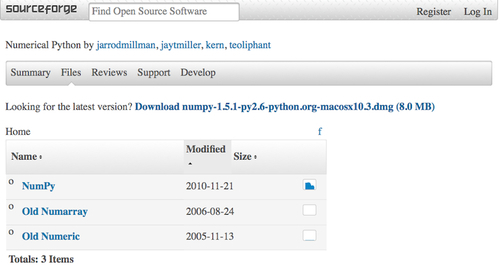2. Open the DMG file : Open the DMG file (in this example, `numpy-1.5.1-py2.6-python.org-macosx10.3.dmg`):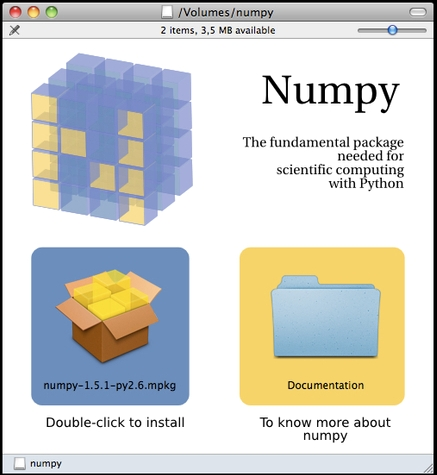• Double-click on the icon of the opened box, the one having a subscript that ends with .mpkg. We will be presented with the welcome screen of the installer.

• Click on the Continue button to go to the ReadMe screen, where we will be presented with a short description of NumPy: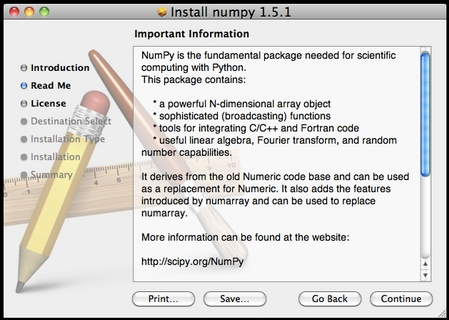• Continue to the License screen.

3. Accept the license: Read the license, click Continue and then the Accept button, when prompted to accept the license. Continue through the next screens and click Finish at the end.

### What just happened?

We installed NumPy on Mac OS X with a GUI installer.

## Time for action – installing NumPy with MacPorts or Fink

Alternatively we can install NumPy through the MacPorts route. It is shown as follows:

1. Installing with MacPorts: Type the following command:

```sudo port install py-numpy
```
2. Installing with Fink: Fink also has packages for NumPy—`scipy-core-py24`, `scipy-core-py25`, and `scipy-core-py26`. We can install the one for Python 2.6 with the following package:

```fink install scipy-core-py26
```

### What just happened?

We installed NumPy on Mac OS X with MacPorts and Fink.

## Building from source

We can retrieve the source code for NumPy with `git`. This is shown as follows:

```git clone git://github.com/numpy/numpy.git numpy
```

Install `/usr/local` with the following command:

```python setup.py buildsudo python setup.py install --prefix=/usr/local
```

To build, we need a C compiler such as GCC and the Python header files in the `python-dev` or `python-devel` package.

## Vectors

NumPy arrays are more efficient than Python lists, when it comes to numerical operations. NumPy code requires less explicit loops than equivalent Python code.

## Time for action – adding vectors

Imagine that we want to add two vectors called a and b. Vector a holds the squares of integers 0 to n, for instance, if n = 3, then a = (0, 1, 4). Vector b holds the cubes of integers 0 to n, so if n = 3, then b = (0, 1, 8). How would you do that using plain Python? After we come up with a solution, we will compare it with the NumPy equivalent.

1. Adding vectors using pure Python: The following function solves the vector addition problem using pure Python without NumPy:

```def pythonsum(n):
a = range(n)
b = range(n)
c = []

for i in range(len(a)):
a[i] = i ** 2
b[i] = i ** 3
c.append(a[i] + b[i])

return c```
2. Adding vector susing NumPy: Following is a function that achieves the same with NumPy.

```def numpysum(n):
a = numpy.arange(n) ** 2
b = numpy.arange(n) ** 3
c = a + b
return c```

Notice that `numpysum()` does not need a `for` loop. Also, we used the `arange` function from NumPy that creates a NumPy array for us with integers 0 to n. The `arange` function was imported; that is why it is prefixed with `numpy`.

Now comes the fun part. Remember that it is mentioned in the preface that NumPy is faster when it comes to array operations. How much faster is Numpy, though? The following program will show us by measuring the elapsed time in microseconds, for the `numpysum` and `pythonsum` functions. It also prints the last two elements of the vector sum. Let's check that we get the same answers by using Python and NumPy:

```import sys
from datetime import datetime
import numpy

def numpysum(n):
a = numpy.arange(n) ** 2
b = numpy.arange(n) ** 3
c = a + b
return c

def pythonsum(n):
a = range(n)
b = range(n)
c = []

for i in range(len(a)):
a[i] = i ** 2
b[i] = i ** 3
c.append(a[i] + b[i])

return c

size = int(sys.argv)
start = datetime.now()
c = pythonsum(size)
delta = datetime.now() - start
print "The last 2 elements of the sum", c[-2:]
print "PythonSum elapsed time in microseconds", delta.microseconds
start = datetime.now()
c = numpysum(size)
delta = datetime.now() - start
print "The last 2 elements of the sum", c[-2:]
print "NumPySum elapsed time in microseconds", delta.microseconds```

The output of the program for 1000, 2000, and 3000 vector elements is as follows:

```\$ python vectorsum.py 1000The last 2 elements of the sum [995007996, 998001000]PythonSum elapsed time in microseconds 707The last 2 elements of the sum [995007996 998001000]NumPySum elapsed time in microseconds 171
\$ python vectorsum.py 2000The last 2 elements of the sum [7980015996, 7992002000]PythonSum elapsed time in microseconds 1420The last 2 elements of the sum [7980015996 7992002000]NumPySum elapsed time in microseconds 168
\$ python vectorsum.py 4000The last 2 elements of the sum [63920031996, 63968004000]PythonSum elapsed time in microseconds 2829The last 2 elements of the sum [63920031996 63968004000]NumPySum elapsed time in microseconds 274
```

### What just happened?

Clearly, NumPy is much faster than the equivalent normal Python code. One thing is certain; we get the same results whether we are using NumPy or not. However, the result that is printed differs in representation. Notice that the result from the `numpysum` function does not have any commas. How come? Obviously we are not dealing with a Python list but with a NumPy array. It was mentioned in the preface that NumPy arrays are specialized data structures for numerical data. We will learn more about NumPy arrays in the next chapter.

### Pop Quiz - functioning of arange function

1. What does `arange(5)` do?

• Creates a Python list of 5 elements with values 1 to 5.

• Creates a Python list of 5 elements with values 0 to 4.

• Creates a NumPy array with values 1 to 5.

• Creates a NumPy array with values 0 to 4.

• None of the above.

### Have a go hero – continue the analysis

The program we used here to compare the speed of NumPy and regular Python is not very scientific. We should at least repeat each measurement a couple of times. It would be nice to be able to calculate some statistics such as average times, and so on. Also, you might want to show plots of the measurements to friends and colleagues.

### Tip

Hints to help you can be found throughout this book and in the online documentation and resources listed at the end of this chapter. NumPy has, by the way, statistical functions that can calculate averages for you. I recommend using `matplotlib` to produce plots.

## IPython—an interactive shell

Scientists and engineers are used to experimenting. IPython was created by scientists with experimentation in mind. The interactive environment that IPython provides is viewed by many as a direct answer to Matlab, Mathematica, and Maple. You can find more information, including installation instructions, at: http://ipython.org/

IPython is free, open source, and available for Linux, Unix, Mac OS X, and Windows. The IPython authors only request that you cite IPython in scientific work where IPython was used. Here is the list of features of IPython:

• Tab completion

• History mechanism

• Inline editing

• Ability to call external Python scripts with `%run`

• Pylab switch

The Pylab switch imports all the `Scipy`, `NumPy,` and `Matplotlib` packages. Without this switch, we would have to import every package we need ourselves.

All we need to do is enter the following instruction on the command line:

```\$ ipython -pylab
Python 2.6.1 (r261:67515, Jun 24 2010, 21:47:49)

IPython 0.10 -- An enhanced Interactive Python.
?         -> Introduction and overview of IPython's features.
%quickref -> Quick reference.
help      -> Python's own help system.
object?   -> Details about 'object'. ?object also works, ?? prints more.

Welcome to pylab, a matplotlib-based Python environment.

In : quit()

```

`quit()` or Ctrl + D quits the IPython shell. We might want to be able to go back to our experiments. In IPython, it is easy to save a session for later.

```In : %logstart
Activating auto-logging. Current session state plus future input saved.
Filename       : ipython_log.py
Mode           : rotate
Output logging : False
Raw input log  : False
Timestamping   : False
State          : active

```

Let's say we have the vector addition program that we made in the current directory. We can run the script as follows:

```In : ls

In : %run -i vectorsum.py 1000

```

As you probably remember, 1000 specifies the number of elements in a vector. The -d switch of `%run` starts an `ipdb` debugger with 'c' the script is started. 'n' steps through the code. Typing `quit` at the `ipdb` prompt exits the debugger.

```In : %run -d vectorsum.py 1000
*** Blank or comment
*** Blank or comment
Breakpoint 1 at /Users/ivanidris/Documents/numpyBeginnersGuide/book/ch1code/vectorsum.py:3

```

### Note

Enter c at the `ipdb>` prompt to start your script.

```><string>(1)<module>()

ipdb> c
> /Users/ivanidris/Documents/numpyBeginnersGuide/book/ch1code/vectorsum.py(3)<module>()
2
1---> 3 import sys
4 from datetime import datetime

ipdb> n
> /Users/ivanidris/Documents/numpyBeginnersGuide/book/ch1code/vectorsum.py(4)<module>()
1     3 import sys
----> 4 from datetime import datetime
5 import numpy

ipdb> n
> /Users/ivanidris/Documents/numpyBeginnersGuide/book/ch1code/vectorsum.py(5)<module>()
4 from datetime import datetime
----> 5 import numpy
6

ipdb> quit

```

We can also profile our script by passing the `-p` option to `%run`.

```In : %run -p vectorsum.py 1000
1058 function calls (1054 primitive calls) in 0.002 CPU seconds

Ordered by: internal time

ncallstottimepercallcumtimepercallfilename:lineno(function)
1    0.001    0.0010.0010.001 vectorsum.py:28(pythonsum)
1    0.001    0.001    0.002    0.002 {execfile}
1000    0.000    0.0000.0000.000 {method 'append' of 'list' objects}
1    0.000    0.000    0.002    0.002 vectorsum.py:3(<module>)
1    0.000    0.0000.0000.000 vectorsum.py:21(numpysum)
3    0.000    0.0000.0000.000 {range}
1    0.000    0.0000.0000.000 arrayprint.py:175(_array2string)
3/1    0.000    0.0000.0000.000 arrayprint.py:246(array2string)
2    0.000    0.0000.0000.000 {method 'reduce' of 'numpy.ufunc' objects}
4    0.000    0.0000.0000.000 {built-in method now}
2    0.000    0.0000.0000.000 arrayprint.py:486(_formatInteger)
2    0.000    0.0000.0000.000 {numpy.core.multiarray.arange}
1    0.000    0.0000.0000.000 arrayprint.py:320(_formatArray)
3/1    0.000    0.0000.0000.000 numeric.py:1390(array_str)
1    0.000    0.0000.0000.000 numeric.py:216(asarray)
2    0.000    0.0000.0000.000 arrayprint.py:312(_extendLine)
1    0.000    0.0000.0000.000 fromnumeric.py:1043(ravel)
2    0.000    0.0000.0000.000 arrayprint.py:208(<lambda>)
1    0.000    0.000    0.002    0.002<string>:1(<module>)
11    0.000    0.0000.0000.000 {len}
2    0.000    0.0000.0000.000 {isinstance}
1    0.000    0.0000.0000.000 {reduce}
1    0.000    0.0000.0000.000 {method 'ravel' of 'numpy.ndarray' objects}
4    0.000    0.0000.0000.000 {method 'rstrip' of 'str' objects}
3    0.000    0.0000.0000.000 {issubclass}
2    0.000    0.0000.0000.000 {method 'item' of 'numpy.ndarray' objects}
1    0.000    0.0000.0000.000 {max}
1    0.000    0.0000.0000.000 {method 'disable' of '_lsprof.Profiler' objects}

```

This gives us a bit more insight in the workings of our program. In addition, we can now identify performance bottlenecks. The `%hist` command shows the commands history.

```In : a=2+2

In : a
Out: 4

In : %hist
1: _ip.magic("hist ")
2: a=2+2
3: a

```

I hope you agree that IPython is a really useful tool!

## Online resources and help

When we are in IPython's pylab mode, we can open manual pages for `NumPy` functions with the help command. It is not necessary to know the name of a function. We can type a few characters and then let tab completion do its work. Let's, for instance, browse the available information for the `arange` function.

```In : help ar<Tab>
arangearccosarccosharcsinarcsinh
arctan        arctan2       arctanhargmaxargmin
argsortargwhere      around        array2string  array_equal
array_equivarray_reprarray_splitarray_str     arrow
array

In : help arange

```

Another option is to put a question mark behind the function name.

```In : arange?
```

The main documentation website for NumPy and SciPy is at http://docs.scipy.org/doc/. Through this webpage, we can browse the NumPy reference at http://docs.scipy.org/doc/numpy/reference/ and the user guide as well as several tutorials.

NumPy has a wiki with lots of documentation at http://docs.scipy.org/numpy/Front%20Page/.

The NumPy and SciPy forum can be found at http://ask.scipy.org/en.

The popular Stack Overflow software development forum has hundreds of questions tagged "numpy". To view them, go to http://stackoverflow.com/questions/tagged/numpy.

If you are really stuck with a problem or you want to be kept informed of NumPy development, you can subscribe to the NumPy discussion mailing list. The e-mail address is . The number of e-mails per day is not too high and there is almost no spam to speak of. Most importantly, developers actively involved with NumPy also answer questions asked on the discussion group. The complete list can be found at http://www.scipy.org/Mailing_Lists.

For IRC users, there is an IRC channel on `irc.freenode.net`. The channel is called #scipy , but you can also ask NumPy questions since SciPy users also have knowledge of NumPy, as SciPy is based on NumPy. There are at least 50 members on the scipy channel at all times.

## Summary

In this chapter, we installed NumPy. We got a vector addition program working and convinced ourselves that NumPy has superior performance. We were introduced to the IPython interactive shell. In addition, we explored the available NumPy documentation and online resources.

In the next chapter, we will take a look under the hood and explore some fundamental concepts including arrays and data types.